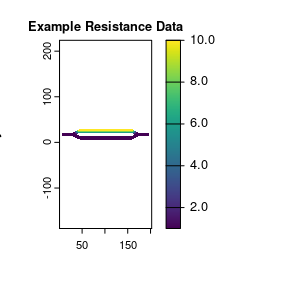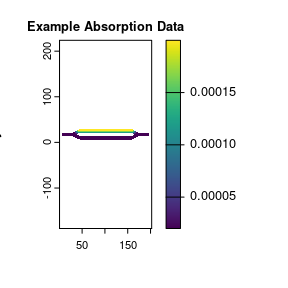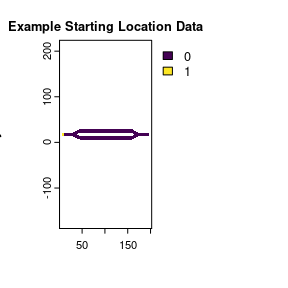# Overview

## Background

This package provides a series of functions for working with absorbing Markov chains. A Markov chain is a model consisting of multiple states and describes how transitions occur between these states. An absorbing Markov chain is a special kind of Markov chain where every state is a transient state that can eventually reach one or more absorbing states. Absorbing states are a special type of state that cannot be left. Absorbing Markov chains can be represented using a $$P$$ matrix with the following structure:

$P = \begin{bmatrix} Q & R \\ 0 & I \end{bmatrix}$ Where:

• $$Q$$ is a matrix that describes the probability of transition between different transient states. $$Q_{i,j}$$ is the probability of transitioning from transient state $$i$$ to transient state $$j$$. When $$i=j$$, the transition probability is describing the probability that a transient state stays the same or doesn’t transition to a different transient state.
• $$R$$ is a matrix that describes the probability of transitioning from a transient state to an absorbing state. $$R_{i,k}$$ is the probability of transitioning from transient state $$i$$ to absorbing state $$k$$.
• $$0$$ is a matrix filled with zeros. The rows correspond to absorbing states $$k$$, and the columns correspond to transient states $$j$$. This represents that an absorbing state has a 0% probability of transitioning to a transient state
• $$I$$ is an identity matrix where each row and column corresponds to the different absorbing states. The main diagonal is filled with ones, and everything else is set to zero. This matrix represents that absorbing state $$k$$ has a 100% probability of transitioning to itself (i.e., the state will never change).
• When combined, $$\begin{bmatrix}0 & I\end{bmatrix}$$ represents that once an absorbing state has been entered, it cannot be left for a transient state or a different absorbing state.

## The samc-class

The samc-class is used to manage the $$P$$ matrix and other information to help ensure that the calculations used by the rest of the package are used correctly. Creating a samc-class object is the mandatory first step in the package, and is created using the samc() utility function. When creating the $$P$$ matrix, samc() only treats the $$R$$ matrix portion as a single column containing the total absorption probability for each transient state. The samc() function has several parameters that provide many different options for constructing the $$P$$ matrix that is at the core of the samc-class.

#### Option 1: Maps

The first option is to use a map of resistance (or conductance) and a map of total absorption with a list of transition arguments to calculate the transition probabilities between cells in the maps. There are certain requirements for these maps:

• They must be 2-dimensional matrices, RasterLayer, or SpatRaster objects. These different types cannot be mixed.
• All inputs must have the same dimensions (number of rows and columns).
• NA is allowed in the cells, but must match between the sets of data. I.e., if cell [3, 6] of the resistance data has a NA value, then cell [3, 6] of the absorption data must also have a NA value and vice versa.

If using SpatRaster or RasterLayer objects, then additional conditions must be met:

• Both sets of data must have the same coordinate extents.
• Both sets of data must use the same coordinate reference system (CRS).

An optional fidelity map may be provided. This map would represent the probability of no transition between timesteps (e.g., no movement). By default, the package treats all cells in the maps the same and uses a value of 0 for fidelity. If used, the fidelity map must meet all of the same requirements listed above for the other map inputs.

#### Option 2: P Matrix

The second option for using this package is to directly supply a $$P$$ matrix. The $$P$$ matrix can be provided either as a regular matrix or a dgCmatrix, which is a sparse matrix object available through the Matrix package. The $$R$$ portion of the $$P$$ matrix must be a single column that represents the total absorption probability for each transient state.

The advantage of this approach is total flexibility. The disadvantage is that the $$P$$ matrix can be created with certain properties that would lead to crashes, and the package is unable to detect all of them at this time. The other disadvantage is that the package cannot map the results back to anything for visualization purposes.

#### Option 3: igraph

A future version of the package will incorporate igraph support for graph-based inputs. This will provide the flexibility of a custom $$P$$ matrix, but will generally be more user-friendly to construct, be able to perform more thorough data checking to avoid issues in the $$P$$ matrix, and allow for mapping the results back to a graph for visualization purposes.

## Utility Functions

In addition to the samc() function, the package has other utility functions that users might find helpful:

• The check() function is used to check that input map data meets the data requirements outlined above. It can be used to compare two SpatRaster objects, two RasterLayer objects, two matrix objects, or check either a SpatRaster, RasterLayer, or a matrix against an already created samc-class object. It can also be used with a multilayer SpatRaster or a RasterStack to check all the layers in the stack against one another.
• The map() function is used to simplify mapping vector results based on input maps and returns a object with the same data type that was used to create the samc-class object. This is provided because R handles matrices and raster layers somewhat differently when reading and writing vector data, which can cause users to map the data incorrectly if they aren’t careful. It also handles mapping with NA values, another potential source of error.
• The locate() function is used to get cell numbers for use as origin and dest values in various analytical function arguments. This function should be used instead of cellFromXY() in the raster or terra packages because cellFromXY() cell numbers do not necessarily correspond to cell numbers in the samc package (the samc package does not assign cell numbers to NA cells, whereas other packages do). The locate() function can be used to return a map with the cell numbers encoded as cell values by simply excluding the xy argument. In this case, the map will have the same class type as the inputs to samc()
• The pairwise() function is provided to easily and efficiently run specific metrics for all the pairwise combinations of start and end locations.

## Analytical Functions

The package implements functions for the formulas provided in Table 1 of Fletcher et al. (2019), as well as other new ones since that publication. Many of the formulas are related conceptually and are grouped into single functions with multiple parameter signatures to reduce the number of unique function names needed. Note that the descriptions assume $$\psi$$ contains probabilities (see above). The following descriptions were written in an ecological context; the function reference pages provide mathematically formal descriptions.

Function Equation Description
absorption() $$A = F R$$ Probability of an individual experiencing a specific type of mortality
$$\psi^T A$$ Probability of an individual experiencing a specific type of mortality, given an initial state $$\psi$$
cond_passage() $$\tilde{t} = \tilde{B}_j^{-1}\tilde{F}\tilde{B}_j{\cdot}1$$ Mean first conditional passage time
dispersal() $$\tilde{D}_{jt}=({\sum}_{n=0}^{t-1}\tilde{Q}^n)\tilde{q}_j$$ Probability of an individual visiting a location, if starting at any other location, before or at time t
$$\psi^T\tilde{D}_{jt}$$ Probability of an individual visiting a location, before or at time t, given an initial state $$\psi$$
$$D=(F-I)diag(F)^{-1}$$ Probability of an individual visiting a location
$$\psi^TD$$ Probability of an individual visiting a location, given an initial state $$\psi$$
distribution() $$Q^t$$ Probability of an individual being at a location at time t
$$\psi^TQ^t$$ Probability of an individual being at a location at time t, given an initial state $$\psi$$
mortality() $$\tilde{B}_t = (\sum_{n=0}^{t-1} Q^n) \tilde{R}$$ Probability of an individual experiencing mortality at a location before or at time t
$$\psi^T \tilde{B}_t$$ Probability of an individual experiencing mortality at a location, before or at time t, given an initial state $$\psi$$
$$B = F \tilde{R}$$ Probability of an individual experiencing mortality at a location
$$\psi^T B$$ Probability of an individual experiencing mortality at a location, given an initial state $$\psi$$
survival() $$z=(I-Q)^{-1}{\cdot}1=F{\cdot}1$$ Expected life expectancy of an individual
$${\psi}^Tz$$ Overall life expectancy, given an initial state $$\psi$$
visitation() $$\tilde{F}_t = \sum_{n=0}^{t-1} Q^n$$ Expected number of times an individual visits a location before or at time t
$${\psi}^T \tilde{F}_t$$ Expected number of times an individual visits a location before or at time t, given an initial state $$\psi$$
$$F = (I-Q)^{-1}$$ Expected number of times an individual visits a location
$${\psi}^T F$$ Expected number of times an individual visits a location, given an initial state $$\psi$$

Depending on the combination of inputs used, a function might return a single value, a vector, a matrix, or a list. In some cases, the calculations will be impractical with sufficiently large landscape datasets due to memory and other performance constraints. To work around this, many equations have multiple associated function signatures that allow users to calculate individual portions of the result rather than the entire result. This opens up multiple optimizations that make calculating many of the metrics more practical. More specific details about performance considerations can be found in the Performance vignette.

#### Initial State Data

Several of the analytical functions allow the input of an initial state $$\psi$$ for the Markov chain via the init parameter. The descriptions for these analytical functions assume that values in $$\psi$$ sum to one. When this is the case, $$\psi_i$$ represents the probability that the Markov chain starts in transient state $$i$$.

When the values in $$\psi$$ sum to a value other than one, care must be taken in the interpretation of the results. For example, $$\psi$$ could be used to represent a population of individuals where $$\psi_i$$ represents the number of individuals that start in transient state $$i$$. In this case, the results of the functions using $$\psi$$ aren’t probabilities, but rather the expected number of individuals.

## Built-in Example Data

The package includes built-in example map data. Some of this data was used to create the figures in the SAMC paper and is used in numerous package tutorials.

• example_split_corridor: A list with three matrices
• res: A matrix with landscape resistance data.
• abs: A matrix with landscape absorption (mortality) data.
• init: A matrix with initial starting locations.
str(samc::example_split_corridor)
#> List of 3
#>  $res : num [1:34, 1:202] NA NA NA NA NA NA NA NA NA NA ... #>$ abs : num [1:34, 1:202] NA NA NA NA NA NA NA NA NA NA ...
#>  $init: num [1:34, 1:202] NA NA NA NA NA NA NA NA NA NA ... res_data <- samc::example_split_corridor$res
abs_data <- samc::example_split_corridor$abs init_data <- samc::example_split_corridor$init

plot(rasterize(res_data), main = "Example Resistance Data", xlab = "x", ylab = "y", col = viridis(256))
plot(rasterize(abs_data), main = "Example Absorption Data", xlab = "x", ylab = "y", col = viridis(256))
plot(rasterize(init_data), main = "Example Starting Location Data", xlab = "x", ylab = "y", col = viridis(256))